The rate of change of displacement of an object in a particular direction is called its velocity.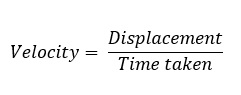Its unit is m/s. Its dimensional formula is [M0 T-1].
It is a vector quantity, as it has both, the magnitude and direction. The velocity of an object can be positive, zero and negative.

## Uniform Velocity:

If an object undergoes equal displacements in equal intervals of time, then it is said to be moving with a uniform velocity.

## Non- Uniform or Variable Velocity:

If an object undergoes unequal displacements in equal intervals of time, then it is said to be moving with a non-uniform or variable velocity.

## Relative velocity:

The relative velocity of one object with respect to another object is the time rate of change of relative position of one object with respect to another object.

Relative velocity of object A with respect to object B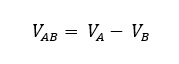When two objects are moving in the same direction, then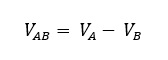When two objects are moving in opposite direction, then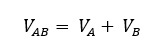Now we consider some special cases:

• If  vB= vA , vB -vA =0, The relative velocity  or  is zero in this case.
• If vB >vA , vA-vB= -ve , is negative.

## Average Velocity:

The ratio of the total displacement to the total time taken is called average velocity.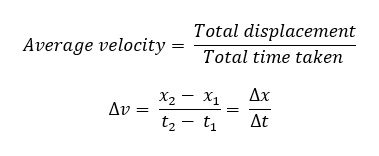The average velocity can be positive or negative depending upon the sign of the displacement. It is zero if the displacement is zero.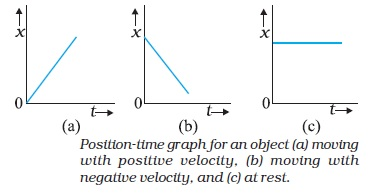## Instantaneous velocity:

The average velocity tells us how fast an object has been moving over a given time interval but does not tell us how fast it moves at different instants of time during that interval. For this, we define instantaneous velocity or simply velocity v at an instant t.

The velocity at an instant is defined as the limit of the average velocity as the time interval Δt becomes infinitesimally small. In other words,